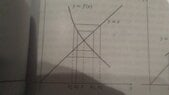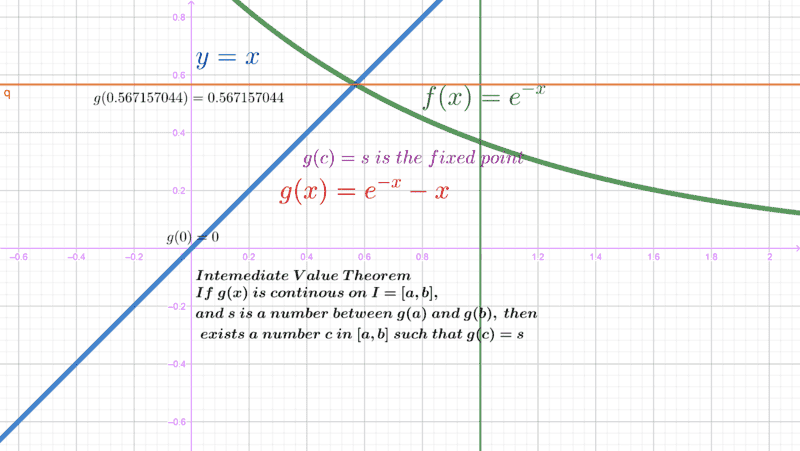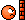# Looking for Guarantees that the method of fixed-point iteration will work

• I
• mcastillo356

#### mcastillo356

Gold Member
TL;DR Summary
Want to understand which kind of functions I can be sure that the method of fixed-point iteration is suitable
Hi PF

Not every function works when we try to compute the root with this methodThe following theorem guarantees that the method of fixed point iteration will work for a particular class of functions

A fixed point theorem
Suppose that ##f## is defined on an interval ##I=[a,b]## and satisfies the following two conditions
(i) ##f(x)## belongs to ##I## whenever ##x## belongs to ##I##; and
(ii) there exists a constant ##K## with ##0<K<1## such that for every ##u## and ##v## in ##I##
$$|f(u)-f(v)|\leq K|u-v|$$
Then ##f## has a unique fixed point ##r## in ##I##, that is, ##f(r)=r##, and starting with any number ##x_0## in ##I##, the iterates
$$x_1=f(x_0),\qquad{x_2=f(x_1),...}$$
converge to ##r##
Hints for the proof
1- Condition (ii) of theorem implies that ##f## is continuous on ##I=[a,b]##. Use condition (i) to show that ##f## has a unique fixed point ##r## on ##I##. Apply the Intermediate-Value Theorem to ##g(x)=f(x)-x## on ##[a,b]##.
2- Use condition (ii) of theorem and mathematical induction to show that ##|x_n-r|\leq K^{n}|x_0-r|##. Since ##0<K<1##, we know that ##K^{n}\rightarrow{0}## as ##n\rightarrow{\infty}##. This shows that ##\lim_{n\rightarrow{infty}}{x_n=r}##
First: ¿Why bounded Newton quotient means continuity on the interval?.

Thanks

Continuity of ##f## on ##I## means that for any ##v\in I## and ##\epsilon >0## we can find ##\delta>0## such that for all ##u\in I## we have ##|f(u)-f(v)|<\epsilon##.

Given ##K,\epsilon## and ##v##, how would you use the inequality given in the OP to find a suitable ##\delta## that satisfies the required condition for continuity?

•mcastillo356 and berkeman
Hi, PF
Sorry, I have had to review all: the concept of limit, and, consequently, continuity. And still have uncertainty though hope to have solved everything: bounded Newton quotient absolute value means continuity, because "a precise definition of limit is based on the idea of controlling the input ##x## of a function ##f## so that the output ##f(x)## will lie in a specific interval. When we say that ##f(x)## has a limit ##L## as ##x## approaches ##a##, we are really saying that we can ensure that the error ##|f(x)-L|## will be less than any allowed tolerance, no matter how small, by taking a ##x## close enough to ##a## (but not equal to ##a##)" (Calculus, 9th edition, by Robert A. Adams and Christopher Essex). These words mean specifically that $$\dfrac{f(u)-f(v)}{u-v}$$ is bounded by ##K##, i.e., we have a limit on the Newton quotient, therefore a derivative, and eventually continuity.

Given ##K,\epsilon## and ##v##, how would you use the inequality given in the OP to find a suitable ##\delta## that satisfies the required condition for continuity?

##\delta=(\epsilon/K)##

Is it ok?

Greetings!

##\delta=(\epsilon/K)##
Yes, that works.

•mcastillo356
Hi
I'm wondering...
I would like to have the written proof itself.
No way?
Greetings

Hi, PF
Sorry, I have had to review all: the concept of limit, and, consequently, continuity.
bounded Newton quotient absolute value means continuity, because "a precise definition of limit is based on the idea of controlling the input ##x## of a function ##f## so that the output ##f(x)## will lie in a specific interval. When we say that ##f(x)## has a limit ##L## as ##x## approaches ##a##, we are really saying that we can ensure that the error ##|f(x)-L|## will be less than any allowed tolerance, no matter how small, by taking a ##x## close enough to ##a## (but not equal to ##a##)" (Calculus, 9th edition, by Robert A. Adams and Christopher Essex). These words mean specifically that $$\dfrac{f(u)-f(v)}{u-v}$$ is bounded by ##K##, i.e., we have a limit on the Newton quotient, therefore a derivative, and eventually continuity.
This last quote is wrong
##f(x)=|x|##. We can make
$$\dfrac{|f(u)-f(v)|}{|u-v|}=\dfrac{||u|-|v||}{|u-v|}\leq 1$$

But this function has not derivative at ##0##

Last edited:
You are correct. The author's statement that we have a derivative is wrong. Fortunately it is not necessary to reach the desired conclusion, which is that ##f## is continuous.

•mcastillo356
Thanks, PF, for editing the right way!

¡Viva!

You are correct. The author's statement that we have a derivative is wrong. Fortunately it is not necessary to reach the desired conclusion, which is that ##f## is continuous.
Just to point out Lipschitz functions * are a.e. differentiable. Maybe this can be used.

* In f.d . spaces.

•mcastillo356
Hints for the proof
1- Condition (ii) of theorem implies that ##f## is continuous on ##I=[a,b]##. Use condition (i) to show that ##f## has a unique fixed point ##r## on ##I##. Apply the Intermediate-Value Theorem to ##g(x)=f(x)-x## on ##[a,b]##.
Hi, PF, now that we know ##f## is continuous on ##[a,b]##, next step, i,e., next two sentences of the quote above.
1- Use condition (i) to achieve the first hint
Condition (i), if I am right, is ##Dom(f)\subseteq{Im(f)}##. This is the tool to show ##f## has a fixed point on ##I=[a,b]##. We must apply the IVT to ##g(x)=f(x) -x##, this is, some ##x=c## in the interval must be ##g(c)=0##. But this is not IVT, is Bolzano's Theorem, to be precise.
Question: What means exactly ##Dom(f)\subseteq{Im(f)}##?
Regards

Hi, PF, now that we know ##f## is continuous on ##[a,b]##, next step, i,e., next two sentences of the quote above.
1- Use condition (i) to achieve the first hint
Condition (i), if I am right, is ##Dom(f)\subseteq{Im(f)}##. This is the tool to show ##f## has a fixed point on ##I=[a,b]##. We must apply the IVT to ##g(x)=f(x) -x##, this is, some ##x=c## in the interval must be ##g(c)=0##. But this is not IVT, is Bolzano's Theorem, to be precise.
Question: What means exactly ##Dom(f)\subseteq{Im(f)}##?
Regards
I assume ##Dom(f)\subseteq{Im(f)}## means set inclusion. The standard proof of this theorem I'm aware of, iterates the contraction map.

•mcastillo356
Condition (i), if I am right, is ##Dom(f)\subseteq{Im(f)}##. ...
'''
What means exactly ##Dom(f)\subseteq{Im(f)}##?
Regards
Where did you get that condition from? It's wrong. Counterexample: the constant mapping ##f(x)= 0##. Then ##Dom(f)=[0,1]\not\subseteq Im(f) = \{0\}##.
It should be the other way around: ##Im(f)\subseteq{Dom(f)}##

•mcastillo356
Where did you get that condition from? It's wrong. Counterexample: the constant mapping ##f(x)= 0##. Then ##Dom(f)=[0,1]\not\subseteq Im(f) = \{0\}##.
It should be the other way around: ##Im(f)\subseteq{Dom(f)}##
Ok, restart with an easy exercise. Find a root of the equation ##x=e^{-x}##. I play tricks graphing ##g(x)=e^{-x}-x##, just to find out ##x_0=1##. Twenty iterations lead me to ##r=0.567157044##, ##\mbox{error}<10^{-4}##.
Now let's leave behind this weird example to talk about ##f(x)=e^{-x}##. What means ##Im(f)\subseteq{Dom(f)}##? ##[0,1]\subseteq{[0,1]}##?

The following theorem guarantees that the method of fixed point iteration will work for a particular class of functions

A fixed point theorem
Suppose that ##f## is defined on an interval ##I=[a,b]## and satisfies the following two conditions
(i) ##f(x)## belongs to ##I## whenever ##x## belongs to ##I##; and
(ii) there exists a constant ##K## with ##0<K<1## such that for every ##u## and ##v## in ##I##
$$|f(u)-f(v)|\leq K|u-v|$$
Hints for the proof
1- Condition (ii) of theorem implies that ##f## is continuous on ##I=[a,b]##. Use condition (i) to show that ##f## has a unique fixed point ##r## on ##I##. Apply the Intermediate-Value Theorem to ##g(x)=f(x)-x## on ##[a,b]##.
We have proved ##f## is continuous on ##I=[a,b]##. Now, condition (i), i.e., ##Im(f)\subseteq{Dom(f)}##.
Question
Why if I apply IVT to ##g(x)=f(x)-x## on ##[a,b]##, provided ##Im(f)\subseteq{Dom(f)}##, I show that ##f## has got a fixed point?

PS: If I'm asking too much, ignore me, please. Just feel free to answer the way you like. PF, if LaTeX mistakes, sorry.

Regards!

Write some inequalities showing the range of possible values for g(0) and g(1), using the premise assumption (i) that f(x) is in I.
Then consider cases.
If g(0) or g(1) is zero, you've found the fixed point.
If both are nonzero, you will find you can apply the IVT to g on I to prove the existence of a fixed point on I where g is zero.

•mcastillo356
I think this is what the textbook suggests, for ##x=e^{-x}##. I've done it quickly: up the image, there should appear ##g(1)=-0.632120558##. I think the ##I-VT## is the key to get the fixed pointWrite some inequalities showing the range of possible values for g(0) and g(1), using the premise assumption (i) that f(x) is in I.
Then consider cases.
If g(0) or g(1) is zero, you've found the fixed point.
If both are nonzero, you will find you can apply the IVT to g on I to prove the existence of a fixed point on I where g is zero.
Intermediate-Value Theorem applied to ##g(x)=f(x)-x##,
If ##g## is continuous on ##[a,b]## and ##s## is a real number lying between the numbers ##g(a)## and ##g(b)##, then there exists a point ##c## in ##[a,b]## such that ##g(c)=s##.
1- ##g## is continuous on ##[a,b]## because ##f(x)## and ##x## are
2- ##x\in{[a,b]}\Rightarrow{f(x)\in{[a,b]}}##
3- Hypothesis: ##g(a)<s<g(b)## (the same if ##g(b)<s<g(a)##):
4- ##\exists{c}## in ##[a,b]## such that ##g(c)=s=f(c)-c##
5- ##f(a)-a<s<f(b)-b##, on ##[a,b]##
6- ##f(b)-b<s<f(a)-a##, on ##[a,b]##
7-##\therefore{s=0\Rightarrow{\exists{c}}}## such that ##f(c)=c## on ##[a,b]##

Is it ok?
Regards!

You make a hypothesis in step 3, and later steps depend on that hypothesis (or its alternative, in parentheses) being true. So you need to show that that hypothesis or its alternative is true. You have not shown that. In fact you need to show that either:
- the hypothesis is true, or
- its alternative is true, or
- g(a) = 0, or
- g(b) = 0
Also, you should fix s=0 at the hypothesis step, not leave it an unfixed variable.

•mcastillo356
Hi, @andrewkirk , PF
First of all forgive this twist. I will try to put it other way. ResetAims:
1) Justify ##g(x)## is continuous on ##[a,b]##. Already proved
2) If ##g(a)=0## or ##g(b)=0##, check we already have the fixed point. Easy work
3) Otherwise, realize ##g(a)## and ##g(b)## got different signs, and apply Bolzano. A root of ##g(x)## satisfies ##f(c)-c=0##

If ##g(a)\neq 0##, and ##g(b)\neq 0##... Here comes that, if ##f(I)\subseteq{I}##, we conclude ##f(a)\geq a## and ##f(b)\leq b##. Thus, applied this to ##g(x)=f(x)-x##, ##g(a)\geq 0## and ##g(b)\leq 0##. Bolzano theorem: there exists ##\delta\in{[a,b]}## such that ##g(\delta)=\delta##

This is the solution I've been given outlined, but, why if ##f(I)\subseteq{I}##, we conclude ##f(a)\geq a## and ##f(b)\leq b##?

My attempt: the image of ##f## in ##[a,b]## is a subset of the domain of ##f##: ##f(a)## preceeds ##a##, and ##f(b)## stands before ##b##.

Am I on the way?
Love.

##I## is defined as ##[a,b]## which is defined as ##\{x\in\mathbb R\ :\ a\le x\le b\}##.
So saying ##f(a)## is in ##I## is just another way of saying that ##f(a)## is real and ##f(a)\ge a## and ##f(a)\le b##. Similarly for ##f(b)##.

•mcastillo356
Hi, @andrewkirk , PF
Back to #1, just to review done work, and work to be done:
1) Already shown that ##f## has a unique fixed point ##r## on ##I##
2) Next:
The following theorem guarantees that the method of fixed point iteration will work for a particular class of functions

A fixed point theorem
Suppose that ##f## is defined on an interval ##I=[a,b]## and satisfies the following two conditions
(i) (...), and
(ii) there exists a constant ##K## with ##0<K<1## such that for every ##u## and ##v## in ##I##
$$|f(u)-f(v)|\leq K|u-v|$$
Then ##f## has a unique fixed point ##r## in ##I##, that is, ##f(r)=r##, and starting with any number ##x_0## in ##I##, the iterates
$$x_1=f(x_0),\qquad{x_2=f(x_1),...}$$
converge to ##r##
Hints for the proof
1- (...)
2- Use condition (ii) of theorem and mathematical induction to show that ##|x_n-r|\leq K^{n}|x_0-r|##. Since ##0<K<1##, we know that ##K^{n}\rightarrow{0}## as ##n\rightarrow{\infty}##. This shows that ##\lim_{n\rightarrow{\infty}}{x_n=r}##
How about if I give a try and tackle second hint?: use condition (ii) of theorem and mathematical induction to show that ##|x_n-r|\leq K^{n}|x_0-r|##
Here the idea is that ##r## is the fixed point then ##f(r)=r##, and by (ii)
##|x_1-r|=|f(x_0)-f(r)|\leq K|x_0-r|##
##|x_2-r|=|f(x_1)-f(r)|\leq K|x_1-r|\leq{K\cdot K\cdot{|x_0-r|}}=K^2|x_0-r|##
...
This idea is not mine: thanks to Spanish Rincón Matemático.
P.S. I will leave the effort to solve ##x=exp(-x)## for some other thread.
Thanks, love.
Again, PF, check the LaTeX, I will post not previewing. Regards

Hi, @andrewkirk , PF
Back to #1, just to review done work, and work to be done:
1) Already shown that ##f## has a unique fixed point ##r## on ##I##
2) Next:

How about if I give a try and tackle second hint?: use condition (ii) of theorem and mathematical induction to show that ##|x_n-r|\leq K^{n}|x_0-r|##
Here the idea is that ##r## is the fixed point then ##f(r)=r##, and by (ii)
##|x_1-r|=|f(x_0)-f(r)|\leq K|x_0-r|##
##|x_2-r|=|f(x_1)-f(r)|\leq K|x_1-r|\leq{K\cdot K\cdot{|x_0-r|}}=K^2|x_0-r|##
...
Hi, PF, here is my attempt:

Required to prove

##|x_n-r|\leq K^n |x_0-r|##

Let's test for ##n=1## and ##n=2##

##|x_1-r|=|f(x_0)-f(r)|\leq K|x_0-r|##

##|x_2-r|=|f(x_1)-f(r)|\leq K|x_1-r|\leq K\cdot K\cdot |x_0-r|=K^2|x_0-r|##

Assume for ##n=s##

##|x_s-r|\leq K^s |x_0-r|## for ##s\in\Bbb {Z}^+##

And prove

##|x_{s+1}-r|=|f(x_s)-f(r)|\leq K^{s+1}|x_s-r|##

but ##s\in\Bbb {Z}^+\therefore{K^{s+1}<K^s}##

Thus

##|x_{s+1}-r|=|f(x_s)-f(r)|\leq K^{s+1}|x_s-r|\leq K^s|x_s-r|##

##\therefore## By PMI, the statement is true for ##n=1,2,3,...##

Sorry, all my background has been a tutorial on the principle of mathematical induction. I post just to pick your opinions.

Questions:
Is there something usable in my soliloquy?
Any suggestion?

Your attempted proof is mostly correct but you've omitted steps in the last line of inequalities. Here it is with the missing steps inserted:
$$|x_{s+1}-r|=|f(x_s)-f(r)| \leq K|x_s - r| \leq K \cdot K^s|x_0-r| = K^{s+1}|x_0-r|$$

•mcastillo356
When I need to solve an equation like $$f(x)=0,$$ and I can't find a fixed point iteration function that works, I try to see the equation as the minimization problem $$0=\min_{x}g(x),\qquad g(x)=\frac{1}{2}\|f(x)\|^2.$$
Then I can try to solve it by using some numerical methods to handle with the differential equation $$\left\{\begin{array}{llll}v'(t)&=& -\nabla g(v(t))\\ v(0)&=&v_0\end{array}\right.,$$ which is the same as the equation $$\left\{\begin{array}{llll}v'(t)&=& -[Jf(v(t))]^*f(v(t))\\ v(0)&=&v_0\end{array}\right..$$

If you have some guarantee that the Jacobian matrix ##Jf(x)## of ##f(x)## has an inverse, the equation $$\left\{\begin{array}{rrl}J{ f}({ u}(t)){ u}'(t)&=&-\alpha { f}({ u}(t))\\ { u}(0)&=&{ u}_0\end{array}\right.,$$ and the Euler method can produce the Newton method, for instance.

Try to search for gradient descent method on SearchOnMath.

Last edited:
•mcastillo356